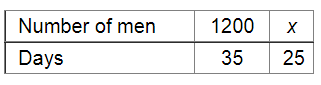# 1200 men can finish a stock of food in 35 days.`
Question:

1200 men can finish a stock of food in 35 days. How many more men should join them so that the same stock may last for 25 days?

Solution:Let x be the number of additional men required to finish the stock in 25 days.

Since the number of men and the time taken to finish a stock are in inverse variation, we have:

$1200 \times 35=25 x$

$\Rightarrow x=\frac{1200 \times 35}{25}$

$=1680$

$\therefore$ Required number of men $=1680-1200=480$

Thus, an additional 480 men should join the existing 1200 men to finish the stock in 25 days.